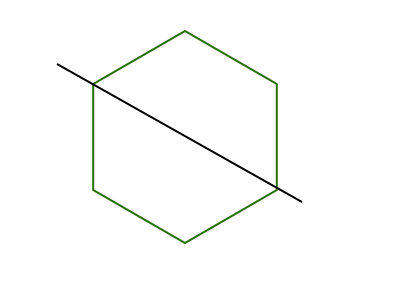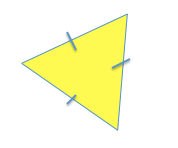MAP 4th Grade Math : Geometry

Example Questions

Example Question #1 : Map 4th Grade Math

The triangle below can be classified as what type of triangle?Equilateral triangle

Scalene triangle

Right triangle

Isosceles triangle

Isosceles triangle

Explanation:

This is an isosceles triangle because an isosceles triangle has two equal sides.

This triangle is a not scalene triangle because a scalene triangle has side lengths of all different lengths.

This is not an equilateral triangle because an equilateral triangle has to have all sides equal in length.

This is not a right triangle because a right triangle has to have a right angle, an angle that measures.

Example Question #2 : Map 4th Grade Math

Does the black line in the figure represent a line of symmetry?Neither choice is correct

Both choices are correct

No

Yes

Yes

Explanation:

When you are trying to decide if a line represents a line of symmetry you can think about folding the figure across that line. Once you make the fold, do all of the parts line up on top?

If yes, then the line represents a line of symmetry.

If no, then the line does not represent a line of symmetry.This line represents a line of symmetry because if you fold on the black line, then one side would lay on top of the other and the parts would match up.

Example Question #3 : Map 4th Grade Math

The triangle below can be classified as what type of triangle?Scalene triangle

Right triangle

Equilateral triangle

Isosceles triangle

Equilateral triangle

Explanation:

This is an equilateral triangle because the sides are all equal, and an equilateral triangle has to have all sides equal in length.

This triangle is not a scalene triangle because all of the side lengths are not different lengths.

This is not an isosceles triangle because an isosceles triangle has only two equal sides.

This is not a right triangle because a right triangle has to have a right angle, an angle that measures